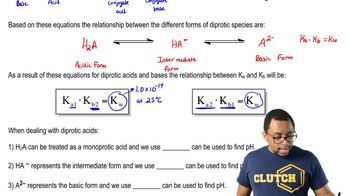Start typing, then use the up and down arrows to select an option from the list.# Diprotic Acids

Jules Bruno
241views
2
now looking at die product basis, We're gonna say the general formula is a to minus because it has a to minus. There, it can accept to h plus is one at a time. Now for those equations, let's take a look. So here we're dealing with the first part of a die product base. It's a to minus form, so it's gonna act as the base so water will be the acid again. Remember, acids donate H plus so that a to minus, except on a H plus to become H A minus water, as a result, becomes O. H minus here. Since we're accepting the first H Plus, we're dealing with K B one are based association constant and just like a one in K two K B one will always be greater than K B two, meaning that it's always easiest to accept that first H plus than it is to accept that second H plus. So here it be H A minus times O. H minus, divided by a two minus. Then, finally, we're gonna say here that we have the possibility of this accepting the second H plus, So if it does, it gives us the second equation here. So again, water is gonna act as the acid. This will be my base. We're gonna donate an H plus. So now it becomes ht way, which is the conjugate acid, all h minus of the conjugate base. So here, up here, this could also be the conjugate acid. And this is the conjugate base again, we're accepting the H plus here for a second time. So we're dealing with baby, too. We ignore solids and liquids. So this is Htwe times of H minus, divided by H A minus. So those were the equilibrium expressions for whether you're dealing with the dye product acid form of the die basic form. Knowing those are key to helping you set up your equilibrium expressions and from there determining your P h and P o h. Now that we've seen, this will continue onward to the last remaining portions when dealing with di product acids and bases03:4302:2304:1413:3406:41